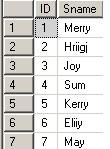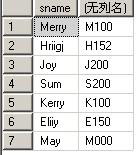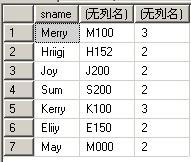# SQL Server中常用字符串函数### 1.len()：计算字符串长度

len()用来计算字符串的长度，每个中文汉字或英文字母都为一个长度

```select sname, len(sname) from student
```

### 2.lower()、upper()：字符串转换为大、小写

lower() 用来将一个字符串转换为小写，upper() 用来将一个字符串转换为大写

```select lower('I AM A STUDENT !')
select upper('i am a student !')
```

### 3.ltrim()、rtrim()：截去字符串左、右侧空格

ltrim()：用来将一个字符串左侧的空格去掉，rtrim()用来将一个字符串右侧的空格去掉。

```declare @str varchar(100);
set @str=' 我的左侧有空格!';
select @str as 初始字符, len(@str) as 初始长度, ltrim(@str) as 现有字符, len(ltrim(@str)) as 现有长度;
```

### 4.space()：返回由重复的空格组成的字符串

space(integer_expression)：integer_expression 指示空格个数的正整数。如果 integer_expression 为负，则返回空字符串。

```select 'A'+ space(2)+'B'    /* 结果： 'A  B' */
```

### 5.substring()、left()、right()：取子字符串

• substring(string, start_position, length)：可以从任意位置取任意长度的子字符串；
• left(string, length)：从左侧开始取子字符串；
• right(string, length)：从右侧开始取子字符串。
```select substring('HelloWorld!', 6, 6)   /* 结果：World */
select left('HelloWorld!', 5)           /* 结果：Hello */
select right('HelloWorld!', 6)          /* 结果：oWorld */
```

### 6.replace()：字符串替换

replace(string, 要被替换的字符串, 替换的字符串)

```select replace('HelloWorld!','o','e')   /* 结果为：HelleWerld! */
```

### 7.reverse()：返回字符串值的逆向值

reverse(string_expression)

```select reverse('abc')   /* 结果为：cba*/
```

### 8.stuff()：删除指定长度的字符，并在指定的起点处插入另一组字符

stuff(character_expression, start, length, character_expression) start：整数，指定删除和插入的开始位置。 length：整数，指定要删除的字符数。

```select stuff('abcdefgf', 2, 6, 'Hello-')    /* 结果为：aHello-f */
select stuff('abcdefgf', 2, 6, 'Hello')        /* 结果为：aHellof */
```

### 9.replicate()：以指定的次数重复字符串值

replicate(string_expression ,integer_expression)

```select replicate('abc', 4)  /* 结果为：abcabcabcabc */
```

### 10.charindex()：返回字符串中指定表达式的开始位置

charindex(expression1 ,expression2 , start_location )或charindex(expression1 ,expression2 ）

expression1在expression2 中的开始位置

```select charindex('H','elloHWorldHabc')    /* 结果为：5 */
```

### 11.patindex()：返回指定“表达式中模式”第一次出现的开始位置

patindex('%pattern%', expression)：返回指定“表达式中某模式”第一次出现的起始位置； 如果在全部有效的文本和字符数据类型中没有找到该模式，则返回零。

```select patindex('%Hello%','WorldHello') 结果为：6
```

### 12.unicode()：返回输入表达式的第一个字符的整数值

unicode('ncharacter_expression')：'ncharacter_expression' 为 nchar 或 nvarchar 表达式。

```select unicode('a')        /* 结果为：97 */
select unicode('abc')    /* 结果为：97 */
```

### 13.str()：返回由数字数据转换来的字符数据

str(float_expression, length ,decimal ) float_expression：带小数点的近似数字 (float) 数据类型的表达式。 length：总长度。它包括小数点、符号、数字以及空格。默认值为 10。 * decimal：小数点右边的小数位数。decimal 必须小于等于 16。如果 decimal 大于 16，则将结果截断为小数点右边的 16 位。

```select str(1234.436, 3)         /* 结果为：'**'；当表达式超出指定长度时返回'**' */
select len(str(1234.436, 3))     /* 结果为：3 */
select str(123.436), len(str(123.436))              /* 结果为：'123', 10 */
select str(123.436, 6), len(str(123.436, 6))        /* 结果为：'123', 6 */
select str(123.436, 6, 1), len(str(123.436,6, 1))   /* 结果为：'123.4', 6 */
```

### 14.ascii()：得到字符的ASCII码

ascii()：用来得到一个字符的ASCII码，它有且只有一个参数，如果参数为字符串，那么取第一个字符的ASCII码

```select ascii('H')               /* 结果为：72 */
select ascii('HelloWorld!')     /* 结果为：72 */
```

### 15.Char()：得到一个与ASCII码数字对应的字符

Char(integer_expression)：integer_expression 介于 0 和 255 之间的整数。如果该整数表达式不在此范围内，将返回 NULL 值。

```select Char(72)     /* 结果为：H */
```

### 16.nchar()：返回返回具有指定的整数代码的 Unicode 字符

nchar(integer_expression) integer_expression 介于 0 与 65535 之间的正整数。如果指定了超出此范围的值，将返回 NULL。

```select nchar(20013)        /* 结果为：'中' */
select NCHAR(72)        /* 结果为：'H' */
```

### 17.soundex()：发音匹配度

```select sname, soundex(sname) from student
``````select sname, soundex(sname), difference(sname,'Herry') from stu
```• 暂无评论